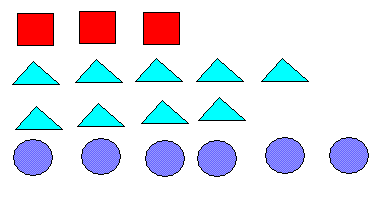Questions on how to find maths ratios in different situations with answers are presented.

## Questions

1. In the figure below are three different shapes: squares, triangles and circles
..

Use the above picture to find the following:
1. the ratio of the number of triangles to the number of circles
2. the ratio of the number of circles to the number of squares
3. the ratio of the number of triangles to total number of shapes
4. the ratio of the number of cirlces to total number of shapes
5. the ratio of the number of circles to number of triangles

2. There are 15 apples, 10 bananas and 5 pears in a basket. Use the given information find the following:
1. the ratio of the number of bananas to the total number of fruits
2. the ratio of the number of pears to the number of apples
3. the ratio of the number of bananas to the number of apples
4. the ratio of the number of pears to the total number of fruits
5. the ratio of the number of apples to the number of fruits

3. In a school there 120 boys and 180 girls. 40 of the boys are under 10 years and 140 of the girls are under 10 years. Use the given information to find
1. the ratio of the number of boys to the number of girls
2. the ratio of the number of boys who are less than 10 to the number of boys who are 10 or older. (in simplest form)
3. the ratio of the number of girls who are less than 10 to the number of boys who are 10 or older. (in simplest form)
4. the ratio of the number of girls who are 10 or older to the total number of pupils. (in simplest form)
5. the ratio of the number of girls who are less than 10 to the total number of pupils who are 10 or older. (in simplest form)

4. a, b and c are the number of blue, yellow and white marbles in a box. a is greater than b and b is greater than c. Answer by true or false the following statements.
1. the ratio of blue marbles to the total number of marbles is less than the ratio of white marbles to the total number of marbles.
2. the ratio of yellow marbles to the total number of marbles is greater than the ratio of blue marbles to the total number of marbles.
3. the ratio of white marbles to the total number of marbles is less than the ratio of blue marbles to the total number of marbles.

1. 9 to 6
2. 6 to 3
3. 9 to 18
4. 6 to 18
5. 6 to 9

1. 10 to 30
2. 5 to 15
3. 10 to 15
4. 5 to 30
5. 15 to 30

1. 2 to 3
2. 1 to 2
3. 7 to 4
4. 2 to 15
5. 7 to 6

1. F
2. F
3. T